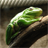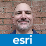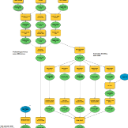# Content of LAYERS.EFLAGS column

2536
7
04-02-2012 01:19 AMNew Contributor
I'm looking for a list with constants to extract information from a value of the LAYERS.EFLAGS column. I know the values for the shape type, but this is not complete. Ineed to read the information about storage type, etc. (SDEBINARY, ST_GEOMETRY).

Where can I find this list of constants?

cu Christian
Tags (3)
7 RepliesbyEsri Esteemed Contributor
The EFLAGS bitmask values are undocumented, and change from release to release.
If there was a list of constants it could be four billion values long.

The information for which you appear to be looking is available elsewhere -- If a layer
is ST_GEOMETRY it will be in the SDE.ST_GEOMETRY_COLUMNS table, and the
SDE.GEOMETRY_COLUMNS table has a STORAGE_TYPE flag (though as a rule, it's
safer to use rows instead of undocumented fields).

- VNew Contributor III

If there was a list of constants it could be four billion values long.

Then the field is poorly named, because that's an enum.  😉

Even allowing a 64-bit field (though it appears to be used as 32-bit) and some grouped bits for e.g. feature type, I'd expect there's less than a hundred.

As a database admin, I'm with Christian that this information, even read-only, could be extremely helpful to analyze and troubleshoot our geodatabase.  Time to put on my engineer hat backwards, I guess….by Anonymous User
Not applicable

Yeah, and because the eflags are undocumented we can only hope for the best when setting this field with sql. And yes we need to.. because registering an empty table registers result in a 2d FC. One workaround would be to insert one 3d geometry in the table before registering, but come on! NO! Another would be using Z and M geoprocessing environment settings in the register tool.byEsri Esteemed Contributor

Directly modifying rows in the SDE-owned tables outside of the stored procedures invoked by ArcObjects is very unsupported. Even if the existing flags were documented, the only supported use would be for read-only metadata purposes. All other uses are likely to result in geodatabase corruption (in the post-9.x denormalized implementation, metadata is redundantly stored for performance optimization; if you miss even one location, integrity is compromised, which could cause upgrade failure years later). I suggest you contact Tech Support for a less risky procedure.

- Vby
New Contributor II

I have the exact same use case.

Registering an existing (Microsoft) SQL table with no records with the geodatabase defaults to a 2D feature. I need this to be 3D. The "Register with Geodatabase" doesn't respect any of the environment settings regarding z-values and also ignores the definition of a vertical coordinate system.

Thank you for the work around of inserting a single 3D record to force detection of a 3D feature. This works partially but introduces issues such as the units for the Z-domain being undefined. It also only pulls the coordinate system from SQL (SRID=2193 is a 2D system) and then prevents any additional assignment of the correct vertical coordinate system (vertical datum) against the data set within the tool UI.

As of 10.8.1 the eflags column in the SDE_layers table seems to be a series of 32 binary flags encoding particular information.

This stackexchange post has determined the first few bits:

• NIL           -> 2^0    (1)

•

POINT         -> 2^1    (2)

•

LINE          -> 2^2    (4)

•

SIMPLELINE    -> 2^3    (8)

•

POLYGON       -> 2^4    (16)

•

MULTIPART     -> 2^18   (262144)

From further investigation it seems the following bit enables the z-values

• 3D Dataset -> 2^16 (65536)

So the following seems to be the minimum required changes the geodatabase metadata for change an empty dataset from 2D to 3D.

Note:

• The @TargetSRID is an existing correctly defined 3D coordinate system within the [SDE_spatial_references] table.
• The name of the database in the example below is 'GIS'
• The script below is only for 3D Point datasets using this value (138739857). The key takeaway is that 65536 needs to be added to the decimal representation of the eflags column to turn on the correct binary flag.

UPDATE
[sde].[SDE_layers]
SET
minz = -1000
,maxz= 10000
,srid=@TargetSRID
,eflags=138739857 --This is binary encoded for a 3d point layer (this is not documented, so not sure what the other flags are for). This should work if this was previously 138674321, the key point is that the number is increasing by 65536
FROM
[sde].[SDE_layers]
WHERE
database_name = 'GIS'
AND [owner] = @FeatureSchema
AND table_name = @FeatureName

UPDATE
sde.GDB_ITEMS
SET
[Definition].modify('declare namespace ns="http://www.w3.org/2001/XMLSchema-instance";
replace value of (/DEFeatureClassInfo/HasZ/text()) with "true"')
FROM
sde.GDB_ITEMS
WHERE
[name] = 'GIS.'+@FeatureSchema+'.'+@FeatureName

UPDATE
sde.SDE_geometry_columns
SET
coord_dimension =3
,srid=@TargetSRID
WHERE
f_table_catalog = 'GIS'
AND f_table_schema = @FeatureSchema
AND f_table_name = @FeatureNameby
New Contributor II

This sql will expand the eflags integer field into the several binary flags

SELECT
convert (varchar(1), eflags / POWER(2,30) % 2)
+ convert (varchar(1), eflags / POWER(2,29) % 2)
+ convert (varchar(1), eflags / POWER(2,28) % 2)
+ convert (varchar(1), eflags / POWER(2,27) % 2)
+ convert (varchar(1), eflags / POWER(2,26) % 2)
+ convert (varchar(1), eflags / POWER(2,25) % 2)
+ convert (varchar(1), eflags / POWER(2,24) % 2)
+ convert (varchar(1), eflags / POWER(2,23) % 2)
+ convert (varchar(1), eflags / POWER(2,22) % 2)
+ convert (varchar(1), eflags / POWER(2,21) % 2)
+ convert (varchar(1), eflags / POWER(2,20) % 2)
+ convert (varchar(1), eflags / POWER(2,19) % 2)
+ convert (varchar(1), eflags / POWER(2,18) % 2)
+ convert (varchar(1), eflags / POWER(2,17) % 2)
+ convert (varchar(1), eflags / POWER(2,16) % 2)
+ convert (varchar(1), eflags / POWER(2,15) % 2)
+ convert (varchar(1), eflags / POWER(2,14) % 2)
+ convert (varchar(1), eflags / POWER(2,13) % 2)
+ convert (varchar(1), eflags / POWER(2,12) % 2)
+ convert (varchar(1), eflags / POWER(2,11) % 2)
+ convert (varchar(1), eflags / POWER(2,10) % 2)
+ convert (varchar(1), eflags / POWER(2,9) % 2)
+ convert (varchar(1), eflags / POWER(2,8) % 2)
+ convert (varchar(1), eflags / POWER(2,16) % 2)
+ convert (varchar(1), eflags / POWER(2,15) % 2)
+ convert (varchar(1), eflags / POWER(2,14) % 2)
+ convert (varchar(1), eflags / POWER(2,13) % 2)
+ convert (varchar(1), eflags / POWER(2,12) % 2)
+ convert (varchar(1), eflags / POWER(2,11) % 2)
+ convert (varchar(1), eflags / POWER(2,10) % 2)
+ convert (varchar(1), eflags / POWER(2,9) % 2)
+ convert (varchar(1), eflags / POWER(2,8) % 2)
+ convert (varchar(1), eflags / 128 % 2)
+ convert (varchar(1), eflags / 64 % 2)
+ convert (varchar(1), eflags / 32 % 2)
+ convert (varchar(1), eflags / 16 % 2)
+ convert (varchar(1), eflags / 8 % 2)
+ convert (varchar(1), eflags / 4 % 2)
+ convert (varchar(1), eflags / 2 % 2)
+ convert (varchar(1), eflags % 2) as [eflagsBinary]
,* from [sde].[SDE_layers]by
New Contributor II

SELECT

POWER(2,29) --is a view -- 536870912

,POWER(2,22) -- is a table  -- 4194304

You can also use SQL bitwise arithmetic operators to test if a flag is set:

SELECT * FROM sde.SDE_layers WHERE eflags & 4 != 0 --All line layers (flag = 4)

SELECT * FROM sde.SDE_layers WHERE eflags & 16 != 0 --All polygon layers (flag = 16)

SELECT * FROM sde.SDE_layers WHERE eflags & 65536 != 0 --All 3d layers (flag = 65536)

SELECT * FROM sde.SDE_layers WHERE eflags & 65552 != 0 --All 3d OR polygon layers (flag 65536 + 16 = 65552)

SELECT * FROM sde.SDE_layers WHERE eflags & 65552 = 65552 --All 3d AND polygon layers (flag 65536 + 16 = 65552)

SELECT * FROM sde.SDE_layers WHERE eflags & POWER(2,29) != 0 --All views

SELECT * FROM sde.SDE_layers WHERE eflags & POWER(2,22) != 0 --All tables

Good explanation of the SQL bitwise arithmetic operator on stack overflow here: https://stackoverflow.com/questions/143712/comparing-two-bitmasks-in-sql-to-see-if-any-of-the-bits-m...